# Volume Scale Factor - Simple Problems

In this worksheet students will be able to find volume of similar shapes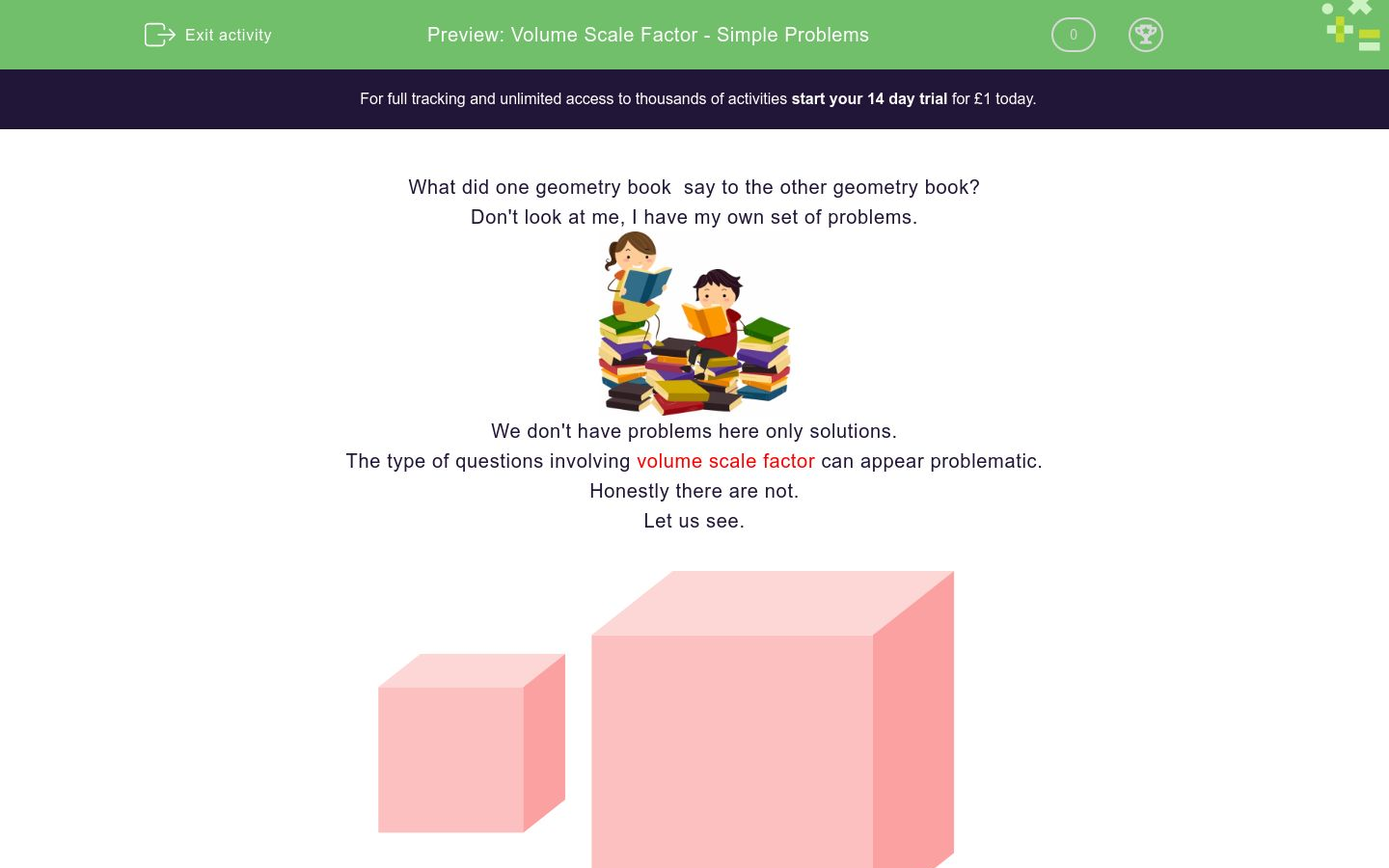Key stage:  KS 4

GCSE Subjects:   Maths

GCSE Boards:   Eduqas, Pearson Edexcel, AQA, OCR

Curriculum topic:   Geometry and Measures, Congruence and Similarity

Curriculum subtopic:   Mensuration and Calculation, Similarity

Difficulty level:### QUESTION 1 of 10

What did one geometry book  say to the other geometry book?

Don't look at me, I have my own set of problems.We don't have problems here only solutions.

The type of questions involving volume scale factor can appear problematic.

Honestly there are not.

Let us see.4 cm                                                            8 cm

Volume  64 cm³                                    What is the volume of this cube?

1.  Find the scale factor of the enlargement    8 ÷ 4  =  Scale factor 2

2.  THIS DOES NOT MEAN THAT THE VOLUME IS TWICE AS BIG.

3.  Take the scale factor and use it as if you were finding the volume i.e 2 x 2 x 2 = 8

4. The volume of the larger cube is 8 times as big

5. 64 x 8  = 512 cm³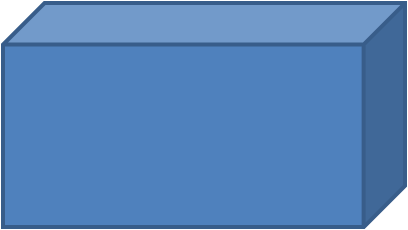6  cm                                                            18 cm

Volume  = 60 cm³                                       What is the volume of this cuboid rectangle?

1.  Find the scale factor of the enlargement    18 ÷ 6  =  Scale factor 3

2.  THIS DOES NOT MEAN THAT THE VOLUME IS THREE TIMES AS BIG.

3.  Take the scale factor and use it as if we were finding a volume. 3 x 3 x 3 = 27

4. The volume of the larger cuboid is 27 times as big

5. 27 x 60  = 1620 cm²

Let us put what we know already into a table

 Scale factor 2 Volume scale factor 2³ 4 times as big Scale factor 3 Volume Scale factor 3³ 27 times as big Scale factor 4 Scale factor 5

Can you spot the pattern and complete the rest of the table?

 Scale factor 2 Volume scale factor 2³ 8 times as big Scale factor 3 Volume scale factor 3³ 27 times as big Scale factor 4 Volume scale factor 4³ 64 times as big Scale factor 5 Volume scale factor 5³ 125 times as big

The volume of our skill set is increasing all the time.

These two prisms are similar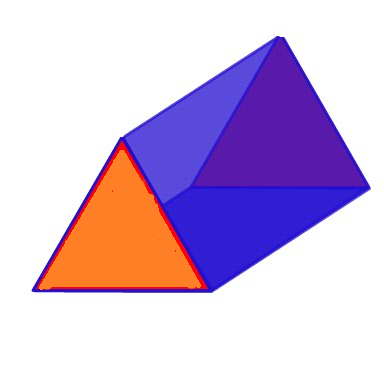Base 3 cm                                                                  Base 9 cm

Volume  =  45 cm²                                                   What is the volume of this prism?

405 cm²

135 cm³

1215 cm³

1456 cm³

These cuboids are similar.  What is the volume of the larger cuboid?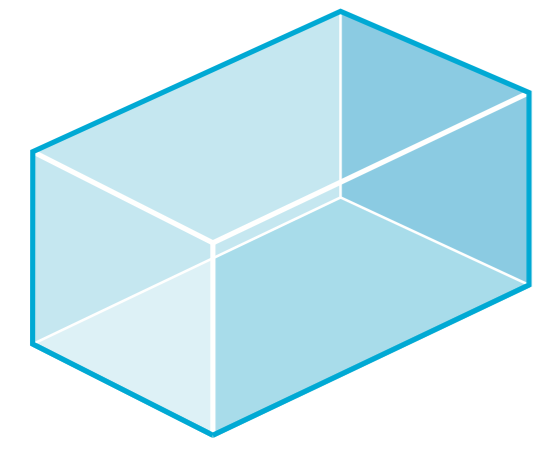Side length 6 cm       Volume  = 96 cm³Side length 24 cm

405 cm²

135 cm³

1215 cm³

1456 cm³Small cube has side of 2 cm, larger cube has side 5 times bigger.

The volume of the smaller cube is 8 cm³

What is the volume of the larger cube?

These two shopping baskets are similar.The smaller basket has side length 8 cm.

The larger basket has a side length of 16 cm.

The volume of the larger basket is 1024 cm³

You are going to cram the larger basket full of chocolate.

Calculate the following:

 124 cm³ 128 cm³ 138 cm³ 906 cm³ 896 cm³ Volume of smaller basket difference in volume of chocolate you can fit into your basket.The volume of water in this swimming pool is 1000 m&sup3;

The length of its side is 20 m.

Another swimming pool has a length of side 30 m.

What is the volume of  water in the other swimming pool?

1500 m³

1750 m³

2750 m³

3375 m²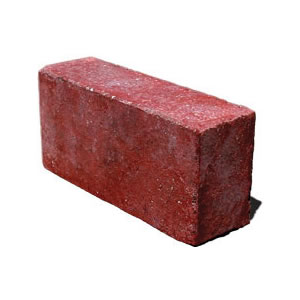These two bricks are mathematically similar.

The larger brick has a side length of 12 cm.  The smaller brick has a side length of 4 cm.

The volume of the larger brick is 144 cm³

Calculate the volume of the smaller brick.

 50.3 cm³ 55.3 cm³ 5.33 cm³ 3.53³ Volume of the smaller brickThere is 330 ml volume of cola is this can?

The can is 6 cm high.

In a promotion another can that is 1.5 times bigger is put on sale.

What is the volume of the cola in the larger can.

1115 cm³

1005 cm³

1255.75 cm³

1113.75 cm³

These two cubes are mathematically similar.

The side of the smaller cube is 4 cm and the side of the larger cube is 12 cm.

The volume of the larger cube is 864 cm³

What is the volume of the smaller cube?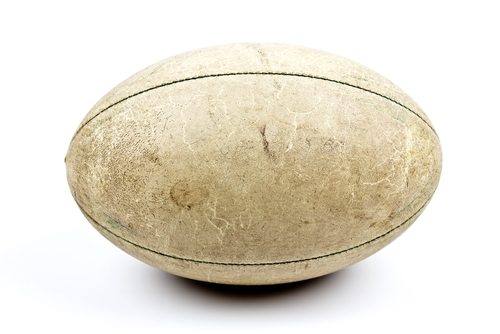This rugby ball has a length of 8 cm and a total volume of 90 cm²

A similar rugby ball has a length of 16 cm.  What is its volume?These two cylinders are mathematically similar.

The radius of the larger cylinder is 15 cm and the radius of the smaller cylinder is 5 cm.

The total volume of the smaller cylinder is 200 cm&sup3;

Calculate the volume of the larger cylinder.

Write you answer in figures without the units.

• Question 1

These two prisms are similarBase 3 cm                                                                  Base 9 cm

Volume  =  45 cm²                                                   What is the volume of this prism?

1215 cm³
EDDIE SAYS
First find the scale factor 9 ÷3 = 3 Find volume scale factor 3 x 3 x 3 = 27 remember it is not 3 times as big The volume of the larger prism is 27 times as big 45 x 27 = 1215 cm³ Thats a lot bigger isn't it.
• Question 2

These cuboids are similar.  What is the volume of the larger cuboid?Side length 6 cm       Volume  = 96 cm³Side length 24 cm

EDDIE SAYS
Scale factor 24 ÷ 6 = 4 Scale factor 4x 4 x 4 = 64 The volume is 64 times larger 96 x 64 = 6144 cm³ Are you getting the hang of cubing the scale factor first?
• Question 3Small cube has side of 2 cm, larger cube has side 5 times bigger.

The volume of the smaller cube is 8 cm³

What is the volume of the larger cube?

140
EDDIE SAYS
Practice is all it takes The question tells us that the scale factor is 5, job done. Volume scale factor is 5 x 5 x 5 = 125 Volume is 125 times as big. 8 x 125 = 1000 cm³ Working with numbers is magic, don't you think?
• Question 4

These two shopping baskets are similar.The smaller basket has side length 8 cm.

The larger basket has a side length of 16 cm.

The volume of the larger basket is 1024 cm³

You are going to cram the larger basket full of chocolate.

Calculate the following:

 124 cm³ 128 cm³ 138 cm³ 906 cm³ 896 cm³ Volume of smaller basket difference in volume of chocolate you can fit into your basket.
EDDIE SAYS
16 ÷ 8 = 2 Volume scale factor is 2³ = 8 This is looking promising, 8 times more chocolate, happy days. Larger basket has a volume of 1024 cm³ Smaller basket has a volume 8 times smaller. 1024 ÷ 8 =128 cm³ 1024 - 128 = 896 cm³ more chocolate for you. Where can I get on of those baskets?
• Question 5The volume of water in this swimming pool is 1000 m&sup3;

The length of its side is 20 m.

Another swimming pool has a length of side 30 m.

What is the volume of  water in the other swimming pool?

3375 m²
EDDIE SAYS
Lets dive into this one. Scale factor 30 ÷ 20 = 1.5 Volume Scale Factor =1.5³ = 3.375 1000 x 3.375 = 3375 m³ Don't let decimals put you off. We deal with them the same way. I am off to cool down now.
• Question 6These two bricks are mathematically similar.

The larger brick has a side length of 12 cm.  The smaller brick has a side length of 4 cm.

The volume of the larger brick is 144 cm³

Calculate the volume of the smaller brick.

 50.3 cm³ 55.3 cm³ 5.33 cm³ 3.53³ Volume of the smaller brick
EDDIE SAYS
Are you building up your skill set now. What looks daunting as a question is not so bad once you can break it down. Scale factor 12 ÷ 4 = 3 Volume scale factor is 3³ = 27 The volume of the small brick is 27 times smaller 144 ÷ 27 = 5.33 cm³ I don't fancy dropping that brick on my foot do you?
• Question 7There is 330 ml volume of cola is this can?

The can is 6 cm high.

In a promotion another can that is 1.5 times bigger is put on sale.

What is the volume of the cola in the larger can.

1113.75 cm³
EDDIE SAYS
This is thirsty work isn't it? We are lucky here because the scale factor has already been given. Volume scale factor is 1.5³ = 3.375 330 x 3.375 = 1113.75 cm³ Cheers
• Question 8

These two cubes are mathematically similar.

The side of the smaller cube is 4 cm and the side of the larger cube is 12 cm.

The volume of the larger cube is 864 cm³

What is the volume of the smaller cube?32
EDDIE SAYS
Scale factor is 12 ÷ 4 = 3 Volume Scale factor is 3³ = 27 Smaller cube has a volume 27 times smaller 864 ÷ 27 = 32 cm³
• Question 9This rugby ball has a length of 8 cm and a total volume of 90 cm²

A similar rugby ball has a length of 16 cm.  What is its volume?

EDDIE SAYS
Scale factor 2 Volume scale factor 2 x 2 x 2 = 8 90 x 8 = 720 cm³ That is a lot of air to get up the dogs nose when he sinks his teeth into it.
• Question 10These two cylinders are mathematically similar.

The radius of the larger cylinder is 15 cm and the radius of the smaller cylinder is 5 cm.

The total volume of the smaller cylinder is 200 cm&sup3;

Calculate the volume of the larger cylinder.

Write you answer in figures without the units.

620
EDDIE SAYS
Scale factor is 15 ÷ 5 = 3 Volume scale factor is 3 x 3 x 3 = 27 200 cm x27 = 5400 cm³
---- OR ----

Sign up for a £1 trial so you can track and measure your child's progress on this activity.

### What is EdPlace?

We're your National Curriculum aligned online education content provider helping each child succeed in English, maths and science from year 1 to GCSE. With an EdPlace account you’ll be able to track and measure progress, helping each child achieve their best. We build confidence and attainment by personalising each child’s learning at a level that suits them.

Get started